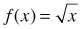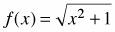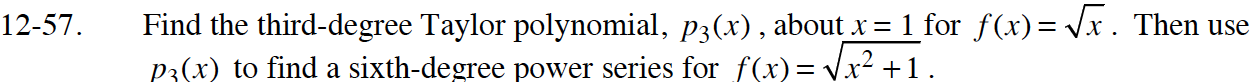### Home > CALC > Chapter 12 > Lesson 12.1.5 > Problem12-57

12-57.

Find the third-degree Taylor polynomial, p3(x), about x = 1 for. Then use p3 (x) to find a sixth-degree power series for. Homework Help ✎$f(x)=x^{1/2},f^\prime(x)=\frac{1}{2}x^{-1/2},f^{\prime\prime}(x)=-\frac{1}{4}x^{-3/2},f^{\prime\prime\prime}(x)=\frac{3}{8}x^{-5/2}$

Simplify.

Substitute x2 + 1 for x into your equation from Step 3 to obtain the sixth-degree power series.